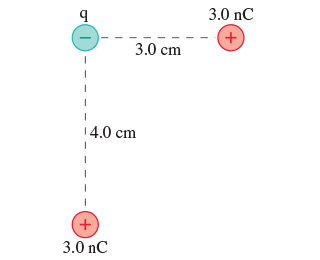In: Physics

# What is the electric potential energy of the group of charges in (Figure 1)? Assume that q = -5.5 nC.

What is the electric potential energy of the group of charges in (Figure 1)? Assume that q = -5.5 nC.U= (value) (units)

## Solutions

##### Expert Solution

Concepts and reason

The concepts used to solve this problem are electric potential energy and Pythagoras theorem. Initially use the Pythagoras theorem to calculate the hypotenuse distance. Then use the pairs of charges and the distance between them in the potential energy formula. Finally add all the potential energy terms to calculate the total potential energy of the group of charges.

Fundamentals

The electric potential energy of a group of charges is,

$$U=\sum_{i, j, i * j} \frac{1}{4 \pi \varepsilon_{0}} \frac{q_{i} q_{j}}{r_{i j}}$$

Here, $$\varepsilon_{0}$$ is the permittivity of the free space, $$q$$ is the charge, and $$r$$ is distance between the two charges, $$U$$ is the

potential energy of the arrangement, and $$i$$ and $$j$$ are indices of charges. Pythagoras theorem gives the hypotenuse distance in a right-angled triangle. $$c=\sqrt{a^{2}+b^{2}}$$

$$a, b,$$ and $$c$$ Here, $$a, b,$$ and $$c$$ are the sides of the triangle.

Use the Pythagoras theorem to find the distance between the two positive charges.

Substitute $$4.0 \mathrm{~cm}$$ for $$a,$$ and $$3.0 \mathrm{~cm}$$ for $$b$$ in the equation $$c=\sqrt{a^{2}+b^{2}}$$

\begin{aligned} c &=\sqrt{(4.0 \mathrm{~cm})^{2}+(3.0 \mathrm{~cm})^{2}} \\ &=\sqrt{16.0 \mathrm{~cm}^{2}+9.0 \mathrm{~cm}^{2}} \end{aligned}

$$=5.0 \mathrm{~cm}$$

Use the potential energy formula.

$$\frac{1}{4 \pi \varepsilon_{0}} \frac{q_{1} q_{2}}{r_{12}}+\frac{1}{4 \pi \varepsilon_{0}} \frac{q_{1} q_{3}}{r_{13}}+\frac{1}{4 \pi \varepsilon_{0}} \frac{q_{2} q_{3}}{r_{23}} \quad \sum_{\text {for } i, j, i * j} \frac{1}{4 \pi \varepsilon_{0}} \frac{q_{i} q_{j}}{r_{i j}}$$ in the equation.

$$U=\sum_{i, j, i * j} \frac{1}{4 \pi \varepsilon_{0}} \frac{q_{i} q_{j}}{r_{i j}}$$

$$U=\frac{1}{4 \pi \varepsilon_{0}} \frac{q_{1} q_{2}}{r_{12}}+\frac{1}{4 \pi \varepsilon_{0}} \frac{q_{1} q_{3}}{r_{13}}+\frac{1}{4 \pi \varepsilon_{0}} \frac{q_{2} q_{3}}{r_{23}}$$

Refer the figure, the three charges make a right-angled triangle at negative charge. There are three charges so

$$1 \leq i, j \leq 3, i \neq j .$$ Here the charge 1 is the bottom positive charge and charge 2 is the negative charge, and charge 3

is the positive charge on the right. The distance between the charge 1 and 2 is $$r_{12}$$. The distance between the charge

2 and 3 is $$r_{23}$$ The distance between the charge 1 and 3 is $$r_{13}$$.

Use the electric potential energy equation.

Substitute $$8.99 \times 10^{9} \mathrm{~N} \cdot \mathrm{m}^{2} / \mathrm{C}^{2}$$ for $$\frac{1}{4 \pi \varepsilon_{0}}, 3.0 \mathrm{nC}$$ for $$q_{1},-5.5 \mathrm{nC}$$ for $$q_{2}, 3.0 \mathrm{nC}$$ for $$q_{3}, 4.0 \mathrm{~cm}$$ for $$r_{12}$$

$$3.0 \mathrm{~cm}$$ for $$r_{23}$$, and $$5.0 \mathrm{~cm}$$ for $$r_{13}$$ in the equation $$U=\frac{1}{4 \pi \varepsilon_{0}} \frac{q_{1} q_{2}}{r_{12}}+\frac{1}{4 \pi \varepsilon_{0}} \frac{q_{1} q_{3}}{r_{13}}+\frac{1}{4 \pi \varepsilon_{0}} \frac{q_{2} q_{3}}{r_{23}}$$

The electric potential energy of the group of charges is $$-7.0 \times 10^{-6} \mathrm{~J}$$.

Calculate the electric potential by substituting the value of charges and distances in the expression of electric potential energy.